Monday , 28 September 2020

# Language of Mathematics

#### How do mathematicians communicate?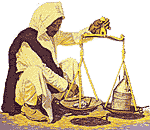You would miss much of the enjoyment of traveling through any new land unless you knew enough of the language to understand what was going on. Communicating mathematical ideas was once a problem even among mathematicians, but they solved this by developing a special language. The next few pages will tell you the meanings of the signs, symbols and words which you need to know to enjoy this book fully.

You already know many of them. Just read them – you do not need to know memorize them. Refer back to these pages whenever you come to know a symbol or word that is unfamiliar. You will soon find that you don’t have to look back very often. Although the language of mathematics many be unfamiliar, it is simple to understand.

#### What is a googol?

If you were walking along the street and found a piece of paper on which was written:

G o o g o l < ∞

Would you know that it is mathematical shorthand? The idea of using shorthand or symbols in a place of words is very old and is widely used. More than 5,000 years ago, ancient Egyptians used symbols to stand for words. Stenographers do it today, although, of course, the symbols are entirely different. Mathematical shorthand is a short and exact way of writing mathematical instructions and quantities.

What does “googol < ∞ ” mean? Translated into everyday English, it reads: a googol is smaller than infinity. The little v turned on its side means is smaller than. Whatever is on the left is smaller than whatever is on the right. The figure , on its side, is the symbol for infinity; that is, a number greater than any we can write, speak or think.

A googol can be written as

10000000000000000000000000000000000000000000000000000000000000000000000000000000000000000000000000000

It is 1 followed by 100 zeros.

It is a number so large that it exceeds the number of raindrops that would fall on London, Manchester and Edinburgh in more than a century. Yet it is smaller than infinity.

Signs and symbols are only one portion of the language of mathematics. Definition of basic terms is another. Together they make up a universal language. In this way, a scientist or mathematicians writing in France or Russia can communicate precisely with his counterpart in the United States or Great Britain. Googol <  has the same meaning to a Canadian as to a Norwegian.

#### What is a prime number?

Numbers are the basic tools of mathematics. If we multiply two or more numbers, we get a new number. For example, 2 x 7 x 11 x 13 = 2,002. The numbers 2, 7, 11 and 13, which we multiplied together, are called factors of 2,002. However, not every number has factors which are whole numbers. Let us take 13. The only numbers, or factors, we can multiply to get 13 are 1 and 13. If a number can not be divided or factored, by any numbers other than itself and 1, that number is called a prime number. How about 11 and 37? Both of these are prime numbers. Is 9 a prime number? No, because it can be factored into 3 x 3 as well as 9 x 1.

#### How does algebra differ from arithmetic?

It has been said that the language of science is mathematics and the grammar of mathematics is algebra. Algebra comes from the Arabic al-jabr, which means the reuniting of broken parts or simplification. Without it, much of our progress would not be possible. Algebra is like a tunnel cut through a mountain – a short cut or the most practical route.

One of the ways in which it differs from arithmetic is that in algebra we use numbers and letters. In algebra, we can say that a case of 50 apples = a. If a man has 10 cases, we write 10a. Note that we omit the multiplication sign between letters and numbers. At all times the letters represent numbers. If we know the quantity, we generally use a, b, c, etc. If the quantity is not known, we usually use x, y or z. Algebra helps us find the value of unknown quantities.

Another basic difference between algebra and arithmetic is the number of fundamental process we use. In arithmetic, we use addition, multiplication, subtraction and division. In algebra, we use these four but also exponents and roots.

Furthermore, in arithmetic, we usually work only with numbers greater than zero, whereas in algebra, we use numbers (or letters that stand for numbers) that are greater or smaller than zero. The numbers that are greater than zero are called positive numbers. Those that are smaller than zero are called negative numbers; they are written with a minus sign in front of them as –x or –43b.

#### What is plane geometry?

Plane geometry is a study of the world around us. It is Greek word which means the measure of land. Without it our buildings would be uneven, our walls would not be perfectly upright, we would be unable to navigate through the air or on the seas. Once you are familiar with the basic language of geometry, you will see how easily everything falls into place.

Let us start with a point. It indicates a position in space. It does not have width, thickness or length.A point in motion produces a line, of which there are several kinds: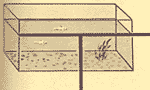A horizontal line is level with the surface of water of water at rest.

A vertical line runs up and down and is at right angles or perpendicular to a horizontal line.An oblique line is neither horizontal nor vertical.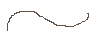A curved line is always changing direction.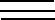Parallel lines are straight lines that never meet no matter how far they are extended.

Angles are formed by two straight lines starting from the same point. Each line is called a side of the angle. The point at which the sides join is called the vertex of the angle.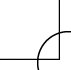One-fourth of a circle forms a right angle. It is ¼ of 360°, or 90°.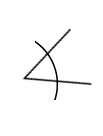An acute angle is any angle smaller then a right angle or 90°.

An obtuse angle is greater than 90°, but is smaller than 180°.A surface is a line in motion. It has both length and width but no depth or thickness. A flat surface, like a desktop, is called a plane surface.

#### What is polygon?

One group of plane surfaces is called polygons. All their sides are straight lines. If all the sides are equal, the figure is then called a regular polygon.

The lowest number of sides which can make a polygon is three. This is a triangle. There are two basic principles which mathematicians have found that apply to all triangles:

The sum of all three angles of a triangles equals 180°.

The length of the side varies according to the size of the angle opposite it. The longest side is opposite the largest angle.

Basically, there are six kinds of triangles:

A right angle triangle has one right or 90° angle. The longest side is opposite this angle.

An isosceles triangles triangle has two equal sides. The angles opposite these sides are also equal.

An acute triangle has all acute angles; that is, each angle is less than 90°.

A scalene triangle has three sides of different lengths.

An obtuse triangle ahs one angle that is greater than 90°.

An equilateral triangle has three equal sides and three equal angles.

Another type of polygon has four side and is called a quadrilateral. The sum of its four angles equals 360°. The sum of the length of its sides is the perimeter.

There are six kinds of quadrilaterals:

A parallelogram has four sides. Each pair of opposite sides are parallel.

A square has four equal sides, and each of its four angles is 90°.

A rectangle is a parallelogram with each of its four angles equal to 90°.

A rhombus has four equal sides. Two of its angles are obtuse, or greater than 90°.

A trapezium has only two sides that are parallel.

A trapezoid has no sides which are parallel.

Polygons can have more than three or four sides. For example, a pentagon has five sides; a hexagon, six; a heptagon, seven; an octagon, eight; and a dodecagon, twelve.

#### What is a circle?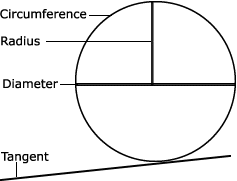As the number of sides increases and approaches infinity, the polygon takes on a new shape. It becomes a circle. A circle is a curved line, every point on which is the same distance from the centre. Every circle has 360°.

The circumference is the outside boundary.

A radius is a straight line from the centre to the circumference.

The diameter is a straight line through the centre of the circle.

A tangent is a straight line, outside the circle, that touches only one point on the circumference.

#### What is solid geometry?When we add thickness to a surface, we leave the realm of plane geometry and enter that of solid geometry.

In this branch of mathematics, we encounter four basic shapes: the sphere, cone, cylinder and polyhedron.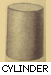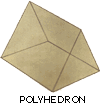Polyhedrons are solids with length, width and thickness. Each surface or face is a polygon. There are only five regular polyhedrons:

A tetrahedron, or pyramid, has four surfaces or faces, each of which is an equilateral triangle.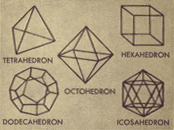A hexahedron, or cube, has six faces, each of which is a square.

An octohedron has eight faces. All the faces are equilateral triangles.

A dodecahedron has twelve faces, each of which is a pentagon.

An icosahedron has twenty faces. All are equilateral triangles.

## साप्ताहिक भविष्यफल अक्टूबर 2020

साप्ताहिक भविष्यफल 27 सितंबर – 03 अक्टूबर, 2020 Weekly Bhavishyafal भविष्यफल अक्टूबर 2020: पंडित असुरारी …